The PANEL Procedure

Panel Data Cross-Sectional Dependence Test

Subsections:

Breusch-Pagan LM Test

Breusch and Pagan (1980) propose a Lagrange multiplier (LM) statistic to test the null hypothesis of zero cross-sectional error correlations. Letbe the OLS estimate of the error term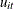under the null hypothesis. Then the pairwise cross-sectional correlations can be estimated by the sample counterparts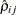,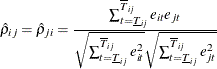where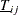and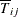are the lower bound and upper bound, respectively, which mark the overlap time periods for the cross sections i and j. If the panel is balanced,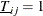and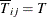. Let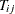denote the number of overlapped time periods (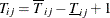). Then the Breusch-Pagan LM test statistic can be constructed as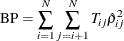When N is fixed and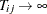,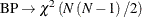. So the test is not applicable as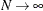.

Because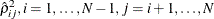, are asymptotically independent under the null hypothesis of zero cross-sectional correlation,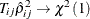. Then the following modified Breusch-Pagan LM statistic can be considered to test for cross-sectional dependence: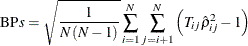Under the null hypothesis,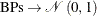as, and then. But because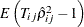is not correctly centered at zero for finite, the test is likely to exhibit substantial size distortion for large N and small.

Pesaran CD and CDp Test

Pesaran (2004) proposes a cross-sectional dependence test that is also based on the pairwise correlation coefficients,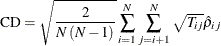The test statistic has a zero mean for fixed N andunder a wide class of panel data models, including stationary or unit root heterogeneous dynamic models that are subject to multiple breaks. For each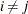, as,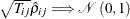. Therefore, for N andtending to infinity in any order,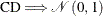.

To enhance the power against the alternative hypothesis of local dependence, Pesaran (2004) proposes the CDp test. Local dependence is defined with respect to a weight matrix,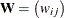. Therefore, the test can be applied only if the cross-sectional units can be given an ordering that remains immutable over time. Under the alternative hypothesis of a pth-order local dependence, the CD statistic can be generalized to a local CD test, CDp,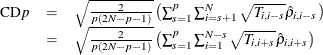where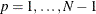. When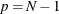, CDp reduces to the original CD test. Under the null hypothesis of zero cross-sectional dependence, the CDp statistic is centered at zero for fixed N and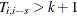, and CD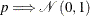asand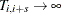.